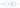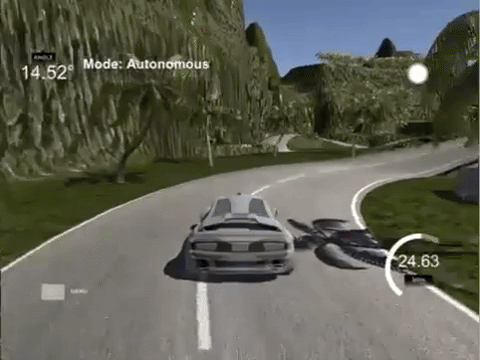📖 You can now grab a copy of our new Deep Learning in Production Book 📖

# Self-driving cars using Deep Learning

Sergios Karagiannakoson2018-09-04·4 mins
Computer VisionSelf-driving cars will be without a doubt the standard way of transportation in the future. Major companies from Uber and Google to Toyota and General Motors are willing to spend millions of dollars to make them a reality, as the future market is predicted to worth trillions. In the past years, we have seen an enormous evolution in the area with cars from Uber, Tesla, Waymo to have a total of 8 million miles in their records.

Of course, self-driving cars are now a reality due to many different technological advancements both in hardware and in software (Spoiler alert: it's Deep Learning). LIDAR sensors, cameras, GPS, ultrasonic sensors are working together to receive data from every possible source. Those data are analyzed in real time using advanced algorithms, making the autopilot functionality possible.

## Steps to program an Autonomous vehicle

There are 5 essential steps to form the self-driving pipeline with the following order:

1. Localization

2. Perception

3. Prediction

4. Planning

5. Control

Localization is basically how an autonomous vehicle knows exactly where it is in the world. In this step, they get the data from all the above-mentioned sensors (sensor fusion) and use a technique called Kalman filters to find their position with the highest possible accuracy. Kalman filter is a probabilistic method that use measurements over time to estimate the state of the object’s position. Another widely used technique is particle filters.

Perception is how cars sense and understand their environment. Here is where computer vision and neural networks come into play. But more on that later.

In the prediction step, cars predict the behavior of every object (vehicle or human) in their surroundings. How they will move, in which direction, at which speed, what trajectory they will follow. One of the most common modes used here is a recurrent neural network, as it can learn from past behavior and forecast the future.

Path planning is self-explainable. It is where that car plans the route to follow or in other words generates its trajectory. This is accomplished with search algorithms (like A*), Lattice planning and Reinforcement Learning.

Finally, control engineers take it from here. They use the trajectory generated in the previous step to change accordingly the steering, acceleration and breaks of the car. The most common method is PID Control but there are a few others such as Linear quadratic regulator(LQR) and Model predictive control(MPC)

Well, I think it’s now time to build an autonomous car by ourselves. Ok, not all of it. But what we can do is use a driving simulator and record what the camera sees. Then we can feed those frames into a neural network and hopefully the car might be able to learn how to drive on its own. Let's see…

We will use Udacity’s open sourced Self-Driving Car Simulator. To use it, you need to install Unity game engine. Now the fun part:

It goes without saying that I spend about an hour recording the frames. It was some serious work guys. I was not fooling around.

Anyway, now the simulator has produced 1551 frames from 3 different angles and also logged the steering angle, the speed, the throttle and the break for each of the different 517 states.

Before we build the model in keras, we have to read the data and split them into the training and test sets.

## Build a Self Driving Car

def load_data():    data_df = pd.read_csv(os.path.join(os.getcwd(),data_dir, 'driving_log.csv'), names=['center', 'left', 'right', 'steering', 'throttle', 'reverse', 'speed'])    X = data_df[['center', 'left', 'right']].values    y = data_df['steering'].values    X_train, X_test, y_train, y_test = train_test_split(X, y, test_size=0.2, random_state=0)    return X_train, X_test, y_train, y_test

After that, we will build our model which has 5 Convolutional, one Dropout and 4 Dense layers.

def build_model():
model = Sequential()    model.add(Lambda(lambda x: x/127.5-1.0, input_shape=INPUT_SHAPE))    model.add(Conv2D(24, kernel_size=(5, 5),strides=(2,2) ,activation='elu'))    model.add(Conv2D(36, kernel_size=(5, 5),strides=(2,2) ,activation='elu'))    model.add(Conv2D(48, kernel_size=(5, 5),strides=(2,2),activation='elu'))    model.add(Conv2D(64, kernel_size=(3, 3), activation='elu'))    model.add(Conv2D(64, kernel_size=(3, 3), activation='elu'))    model.add(Dropout(0.5))    model.add(Flatten())    model.add(Dense(100, activation='elu'))    model.add(Dense(50, activation='elu'))    model.add(Dense(10, activation='elu'))    model.add(Dense(1))    #model.summary()    return model

The network will output only one value, the steering angle.

Before we pass the inputs on the model, we should do a little preprocessing. Note that this is done with OpenCV, an open-sourced library that is build for image and video manipulation.

First of all we have to produce more data and we will do that by augment our existing. We can for example flip the existing images, translate them, add random shadow or change their brightness.

image, steering_angle = choose_image(data_dir, center, left, right, steering_angle)image, steering_angle = random_flip(image, steering_angle)image, steering_angle = random_translate(image, steering_angle, range_x, range_y)image = random_shadow(image)image = random_brightness(image)

Next, we have to make sure to crop and resize the images in order to fit into our network.

def preprocess(image):    image = crop(image)    image = resize(image)    image = rgb2yuv(image)    return image

Training time:

def train_model(model, X_train, X_valid, y_train, y_valid):    model.compile(loss='mean_squared_error', optimizer=Adam(lr=0.001))
#Fits the model on data generated batch-by-batch by a Python generator.    model.fit_generator(batch_generator(data_dir, X_train, y_train, batch_size, True),                        steps_per_epoch,                        num_epochs,                        verbose=1,                        validation_data=batch_generator(data_dir, X_valid, y_valid, batch_size, False),                        validation_steps=40                        )

Now we have the trained model. It has essentially cloned our driving behavior. Let's see how we did it. To do that, we need a simple server (socketio server) to send the model prediction to the simulator in real-time. I am not going to get into many details about the server stuff. What’s important is the part that we predict the steering angle using the frames and logs generated by the simulator in real time.

steering_angle = float(data["steering_angle"])throttle = float(data["throttle"])speed = float(data["speed"])image = Image.open(BytesIO(base64.b64decode(data["image"])))image = np.asarray(image)image = preprocess_data.preprocess(image)image = np.array([image])
steering_angle = float(model.predict(image, batch_size=1))throttle = 1.0 - steering_angle ** 2 - (speed / speed_limit) ** 2
#send prediction to the simulatorsend_control(steering_angle, throttle)

And the result:We actually did it. I think that Udacity’s emulator is the easiest way for someone to start learning about self-driving vehicles. To continue your journey on Autonomous vehicles, I recommend the Self-Driving Cars Specialization by Coursera. It contains everything you need to get started if you are really interested in the field.

To wrap up, autonomous cars have already started being mainstream and there is no doubt that they become commonplace sooner than most of us think. It is extremely complex to build one as it requires so many different components from sensors to software. Deep Learning will definetely play a big role towards this goal. But here we just did a very very small first step.

The major thing is that the future is here. And it is exciting…## Deep Learning in Production Book 📖

#### Learn how to build, train, deploy, scale and maintain deep learning models. Understand ML infrastructure and MLOps using hands-on examples.

* Disclosure: Please note that some of the links above might be affiliate links, and at no additional cost to you, we will earn a commission if you decide to make a purchase after clicking through.